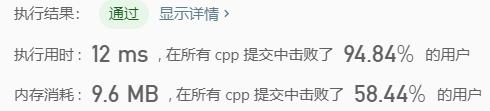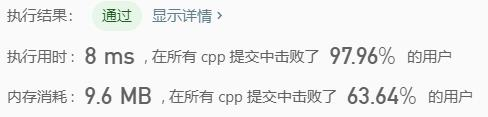# Leetcode 136：只出现一次的数字

## 解题思路

a⊕0=a；a⊕a=0。a⊕b⊕a=(a⊕a)⊕b=0⊕b=b

## 代码实现

int singleNumber(vector<int>& nums) {    //if (nums.empty())    //  return 0;    for (int i = 1; i < nums.size(); i++)        nums ^= nums[i];//异或    return nums;}

## 复杂度## 拓展：Leetcode137（只出现一次的数字2）

### 解题思路

* ones^=num：记录至目前元素num，二进制某位出现1次（当某位出现3次时有ones=1，与twos=1共同表示“出现3次”）。
* twos|=ones&num：记录至目前元素num，二进制某位出现2次（当某位出现2次时，twos=1且ones=0）
* threes=ones&twos：记录至目前元素num，二进制某位出现3次（即当ones和twos对应位同时为1时three=1）。
one&=~threes,two&=~threes：将onestwos中出现了3次的对应位清零，实现“不考虑进位的三进制加法”。

https://leetcode-cn.com/problems/single-number-ii/solution/single-number-ii-mo-ni-san-jin-zhi-fa-by-jin407891/

### 代码实现

int singleNumber(vector<int>& nums) {    //if (nums.empty())    //  return 0;    int ones = 0, twos = 0;    for (int num : nums) {        ones = ones ^ num & ~twos;        twos = twos ^ num & ~ones;    }    return ones;}

### 复杂度分析# Leetcode 139：单词拆分

## 代码实现

bool wordBreak(string s, vector<string>& wordDict) {    int len = s.size();    if (len == 0 || wordDict.size() == 0)        return false;    vector<bool> dp(len + 1, false);    dp = true;    for (int i = 1; i <= len; i++)    {        for (int j = i - 1; j >= 0; j--) {            if (dp[j] && find(wordDict.begin(), wordDict.end(), s.substr(j, i - j)) != wordDict.end())            {                dp[i] = true;                break;            }        }    }    return dp[len];}